# Class 10 RD Sharma Solutions – Chapter 12 Some Applications of Trigonometry – Exercise 12.1 | Set 1

• Last Updated : 20 May, 2021

### Question 1. A tower stands vertically on the ground. From a point on the ground, 20 m away from the foot of the tower, the angle of elevation of the top the tower is 60°. What is the height of the tower?

Solution:

Given that,

The distance between point of observation and foot of tower(BC) = 20 m

Angle of elevation of top of tower(θ) = 60°

And the height of tower (H) = AB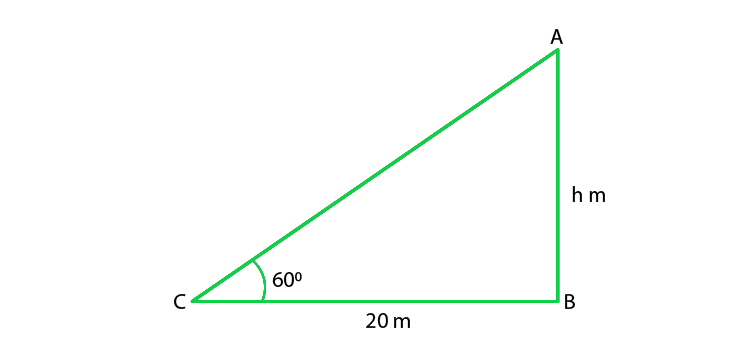From the figure we conclude that ABC is a right angle triangle

So, tanθ = (AB)/(BC)

AB = 20 tan 60o

H = 20 x √3 = 20√3

Hence, the height of tower is 20√3 m

### Question 2. The angle of elevation of a ladder against a wall is 60° and the foot of the ladder is 9.5 m away from the wall. Find the length of the ladder.

Solution :

Given that,

The distance between foot of the ladder and wall(BC) = 9.5 m

Angle of elevation (θ)= 60°

Length of the ladder (L) = AC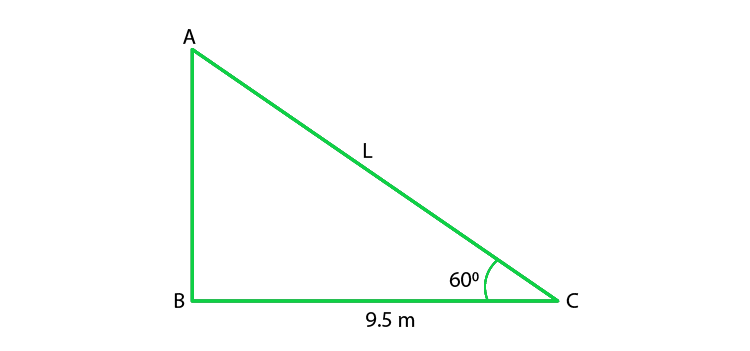From the figure we conclude that ABC is a right angle triangle

So,

cos 60o = BC/AC

AC = 2 x 9.5 = 19m

Hence, the length of ladder is 19m.

### Question 3. A ladder is placed along a wall of a house such that its upper end is touching the top the wall. The foot of the ladder is 2 m away from the wall and the ladder is making an angle of 60° with the level of the ground. Determine the height of the wall.

Solution:

Given that,

The ladder is making an angle of 60°Distance between foot of the ladder and wall(BC) = 2m

Height of the wall (H) = AB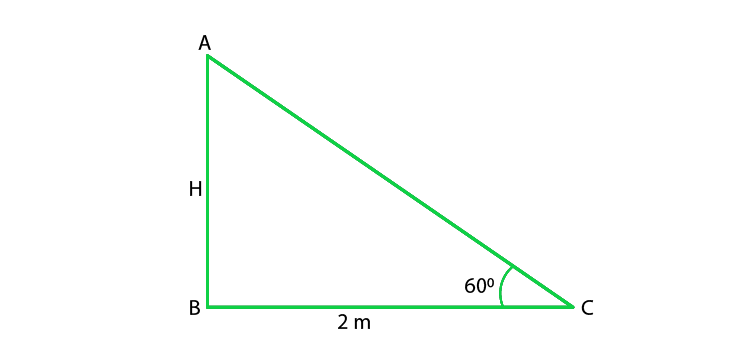From the figure we conclude that ABC is a right angle triangle

So, tan 60o = (AB)/(BC)

√3 = AB/2

AB = 2√3

Hence, the height of wall is 2√3 m

### Question 4. An electric pole is 10 m high. A steel wire to top of the pole is affixed at a point on the ground to keep the pole up right. If the wire makes an angle of 45° with the horizontal through the foot of the pole, find the length of the wire.

Solution: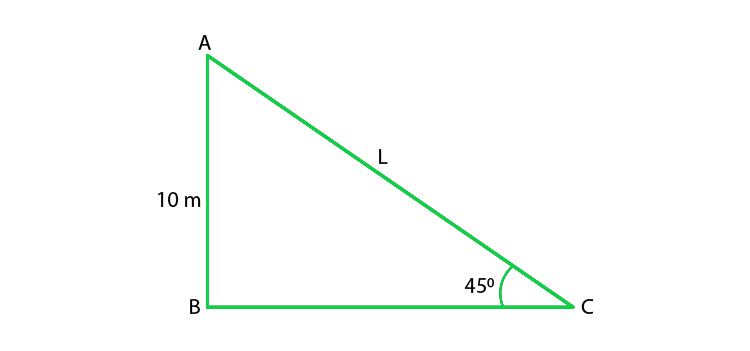Given that,

The height of the electric pole (H) = AB = 10 m

Angle made by steel wire with ground (horizontal) (θ) = 45°

Let us assume length of wire (AC) = L

From the figure we conclude that ABC is a right angle triangle

So,

sin 45o = AB/AC

1/√2 = 10/L

L = 10√2 m

Hence, length of wire is 10√2 m.

### Question 5. A kite is flying at a height of 75 meters from the ground level, attached to a string inclined at 60° to the horizontal. Find the length of the string to the nearest meter.

Solution:

Given that,

The height of kite from ground(AB) = 75 m

Inclination of string with ground (θ) = 60°

And the length of string (L) = AC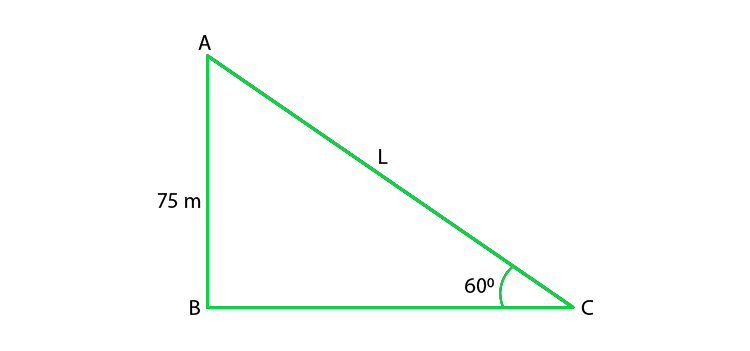From the figure we conclude that ABC is a right angle triangle

So,

sin60o = AB/AC

√3/2 = 75/L

L = 50√3 m

Hence, the length of string is 50√3 m.

### Question 6. The length of a string between a kite and a point on a point on the ground is 90 meters. If the string makes an angle θ with the ground level such that tan θ = 15/8. How high is the kite? Assume that there is no slack in the string

Solution:

Given that,

The length of the string between point on ground and kite = 90 m

Angle made by string with ground is θ

And tan θ =15/8

θ = tan−1(15/8)

Height of the kite be ‘H’ m

So, ABC is a right angle triangle

tanθ = AB/BC

15/8 = H/BC

BC = 8H/15    ———–(i)

In ΔABC,

by using Pythagoras theorem, we get

AC2 = BC2 + AB2

902 = (8H/15)2 + H2

H2 = (90×15)2/289

H = 79.41m

Height of the kite from ground

H = 79.41m

Hence, Height of the kite from ground is 79.43 m

### Question 7. A vertical tower stands on a horizontal place and is surmounted by a vertical flag staff. At a point on the plane 70 meters away from the tower, an observer notices that the angles of elevation of the meters away from the flag staff are respectively 60° and 45°. Find the height of the flag staff and that of the tower.

Solution:

Given that, the vertical tower is surmounted by flag staff.

So, the distance between tower and observer(DC) = 70 m

Angle of elevation of top of tower (α) = 45°

Angle of elevation of top of flag staff (β) = 60°

Height of flag staff (AD) = h

Height of tower(BC) = H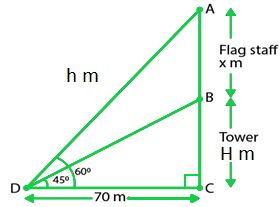From the figure we conclude that ΔACD and ΔBCD are right angle triangles

So,

In ΔBCD,

tanθ = (BC)/(DC)

tan45o = H/70

H = 70

Again, in ΔACD,

tan θ = AC/DC = (x + H)/70

tan 60o = (x + H)/70

√3 = (x + 70)/70

x = 51.24 m

Hence, the height of tower is 70 m and height oh flag staff is 51.24 m

### Question 8. A vertically straight tree, 15 m high; is broken by the wind in such a way that its top just touches the ground and makes an angle of 60° with the ground. At what height from the ground did the tree break?

Solution:

Given that,

The initial height of tree(AB) = H = 15 m

Let us considered that it is broken at point C.

So, the angle made by broken part with the ground (θ) = 60°

And the height from ground to broken points(BC) = h

AB = AC + BC

H = AC + h

AC = (H – h) m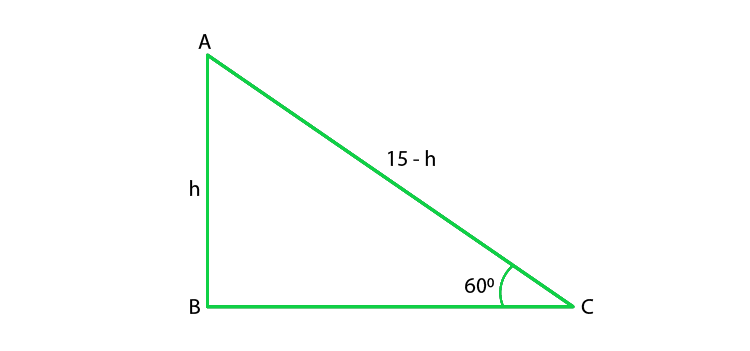From the figure we conclude that ABC is a right angle triangle

So,

sin60o = BC/CA

√3/2 = h/(H – h)

√3(15 – h) = 2h

h = (15√3 / 2 + √3) x (2 – √3 / 2 – √3)

h = 15(2√3 – 3)

Hence, the height of broken points from ground is 15(2√3 – 3) m.

### Question 9. A vertical tower stands on a horizontal plane and is surmounted by a vertical flag staff of height 5 meters. At a point on the plane, the angles of elevation of the bottom and the top of the flag staff are respectively 30° and 60°. Find the height of the tower.

Solution:

Given that,

The height of the flag staff(AB) = h = 5 m

Angle of elevation of the top of flag staff(α) = 60°

Angle of elevation of the bottom of the flagstaff(β) = 60°

Let us assume that the height of tower is (H) = AB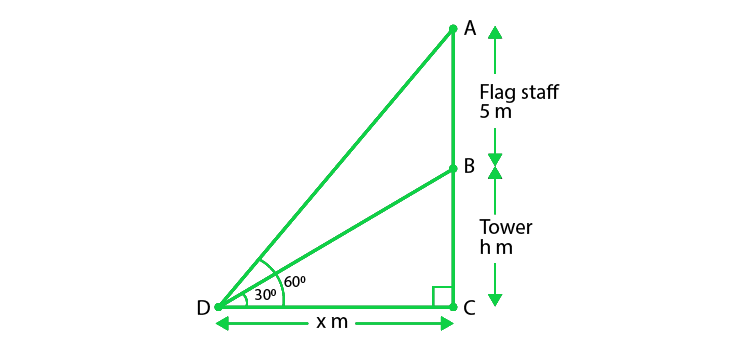From the figure we conclude that ΔACD and ΔBCD are right angle triangles

So, in ΔBCD,

tanθ = (BC)/(DC)

tan60o = h/x

√3 = h/x    —————-(i)

So, in ΔACD,

tanθ = (AC)/(DC)

tan30o = 5 + h/x

1/√3 = 5 + h/x      ——————-(ii)

Equating eqn. (i) and (ii),

h = 2.5 m

Hence, the height of the tower = 2.5 m.

### Question 10. A person observed the angle of elevation of a tower as 30°. He walked 50 m towards the foot of the tower along level ground and found the angle of elevation of the top of the tower as 60°. Find the height of the tower.

Solution:

Given that,

The angle of elevation of top of tour from first point of elevation(α) = ∠A = 30°

Let us considered that the person walked 50 m from first point (A) to (B) then AB = 50 m

Angle of elevation from second point (β) = ∠C = 60°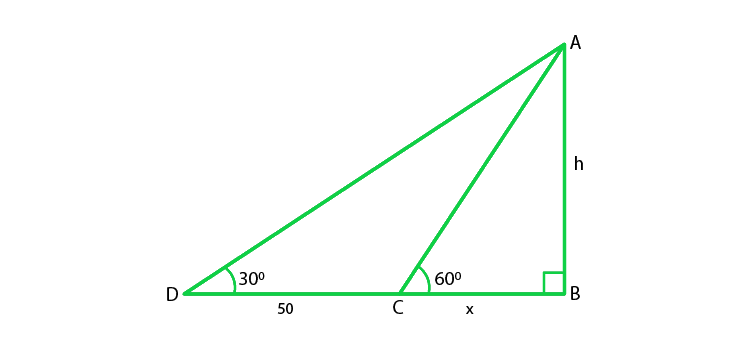From the figure we conclude that ΔABC and ΔABD are right angle triangles

So, ∠B= 90°

Let us considered the height of the tower is (AB) = h

BC = x m.

In ΔABC,

tan θ = AB/BC

tan 60o = h/x

√3 = h/x   ————-(i)

In ΔABD,

tanθ = (AB)/(BD)

tan30o = h/(DC + BC)

1/√3 = h/(50 + x)     ——————–(ii)

From eqn. (i) and (ii), we get

h = 25√3

Hence, the height of tower is 25√3 m

### Question 11. The shadow of a tower, when the angle of elevation of the sun is 45°, is found to be 10 m longer then when it was 60°. Find the height of the tower.

Solution: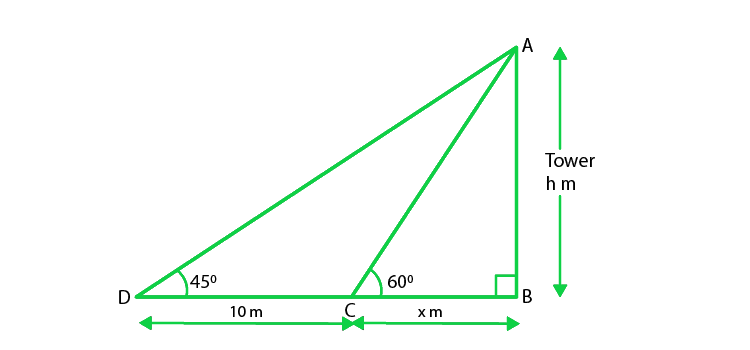Let us considered the length of the shadow of the tower when angle of elevation is 60° be(BC) = x m

Now, the length of the shadow with angle of elevation 45° (BD) = (10 + x)m

Let us assume that the height of the tower(AB) = H

From the figure we conclude that ΔABC and ΔABD are right angle triangles

So, ∠B = 90°

Now, In ΔABC,

tanα = AB/BC

tan60o = H/x

x = H/√3  ——————–(i)

Again, In ΔABD

tanβ = AB/BD

tan45o = H/(x + 20)

x + 10 = H

x = H – 10       ——————–(ii)

From eq(i) and (ii), we get

H – 10 = H/√3

√3 H – 10√3 = H

H = 10√3/(√3 – 1)

H = 5(3 + √3)

Hence, height of tower is 23.66 m.

### Question 12. A parachute is descending vertically and makes angles of elevation of 45° and 60° at two observing points 100 m apart from each other on the left side of himself. Find the maximum height from which he falls and the distance of point where he falls on the ground from the just observation point.

Solution: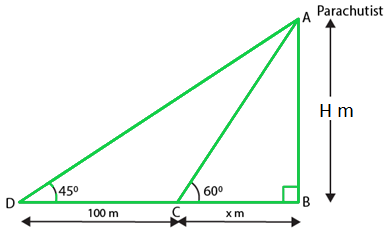Let us considered that the parachute at highest point A.

So, C and D be points which are 100 m apart on ground where from then CD = 100 m

Angle of elevation from point ∠C = 45° = α,

Angle of elevation from point ∠D = 60° = β,

Let’s B be the point just vertically down the parachute

Also, the maximum height of the parachute from the ground (AB) = H m

And the distance of point where parachute falls to just nearest observation point = x m

From the figure we conclude that ΔABC and ΔABD are right angle triangles

Now, in ΔABC

tanα = AB/BC

tan45o = H/x + 100

100+x = H        —————–(i)

In ΔABD

tanβ = AB/BD

tan60o = H/x

H = √3x         ——————(ii)

From eq(i) and (ii), we get

x = 136.6 m

Put the value of x in (ii), we get

H = √3 × 136.6 = 236.6m

Hence, the distance between the two points where parachute falls on

the ground and just the observation is 136.6 m.

### Question 13. On the same side of a tower, two objects are located. When observed from the top of the tower, their angles of depression are 45° and 60°. If the height of the tower is 150 m, find the distance between the objects.

Solution: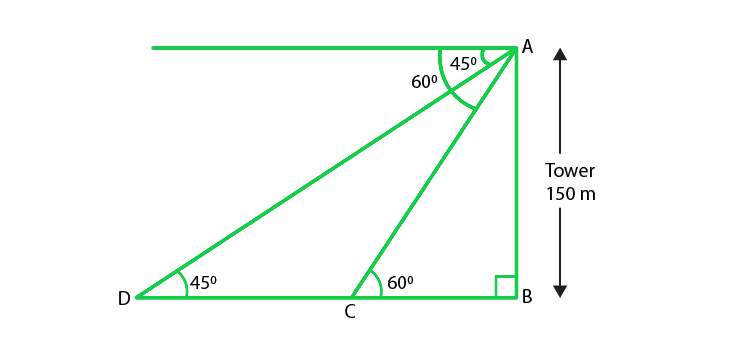Given that, the height of the tower(AB) = H = 150 m

And the angles of depression are 45° and 60°

In ΔABC

tan60° = 150/BC

BC = 150/√3              ——————(i)

Now, In ΔABD

tanβ = AB/BD

tan45° = 150 / BC + CD

BC + CD = 150            —————-(ii)

From eq(i) and (ii), we get

BC = 63.4 m

Hence, the distance between object is 63.4 m.

### Question 14. The angle of elevation of a tower from a point on the same level as the foot of the tower is 30°. On advancing 150 meters towards the foot of the tower, the angle of elevation of the tower becomes 60°. Show that the height of the tower is 129.9 m.

Solution: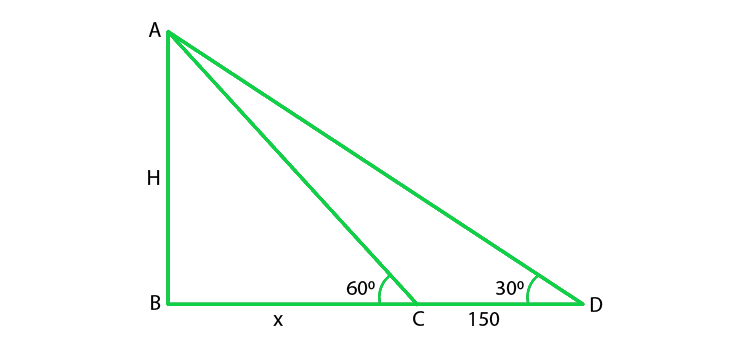Given that, the angle of elevation of top tower from first point D(α) = 30°

CD = 150 m,

Angle of elevation of top of tower from second point C(β) = 60°,

Let us assume that the height of tower AB = H m,

From the figure we conclude that ΔABC and ΔABD are right angle triangles

So, ∠B = 90°,

In ΔABD,

tanα = AB/BD

tan30o = H/(150+x)

150 + x = H√3                  ———————-(i)

Now, in ΔABC,

tanβ = AB/BC

tan60 = H/x

H = x√3                   ————–(ii)

From eq(i) and (ii), we get

H = 129.9 m

Hence, the height of the tower is 129.9 m

### Question 15. The angle of elevation of the top of a tower as observed from a point in a horizontal plane through the foot of the tower is 32°. When the observer moves towards the tower a distance of 100 m, he finds the angle of elevation of the top to be 63°. Find the height of the tower and the distance of the first position from the tower. [Take tan 32° = 0.6248 and tan 63° = 1.9626]

Solution: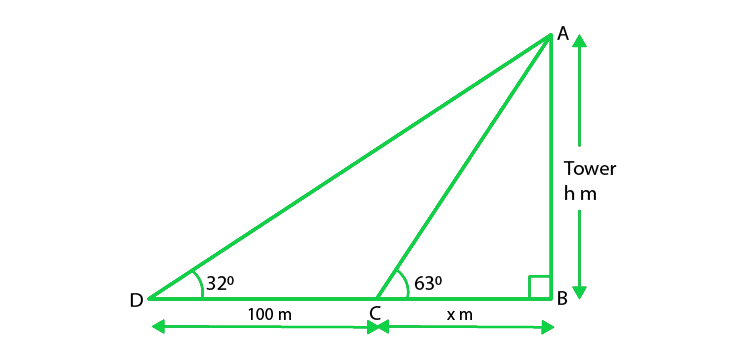Let’ us considered the height of the tower(AB) = h m, and the distance BC = x m

So, in ΔABC

tan 63o = AB/BC

1.9626 = h/x

x = h/ 1.9626

x = 0.5095 h …. (i)

Now, in ΔABD

tan 32o = AB/ BD

0.6248 = h/ (100 + x)

h = 0.6248(100 + x)

h = 62.48 + 0.6248x

h = 62.48 + 0.6248(0.5095 h) ….. [Using eq(i)]

h = 62.48 + 0.3183h

0.6817h = 62.48

h = 62.48/0.6817 = 91.65

Now put the value of h in eq(i), we get

x = 0.5095(91.65) = 46.69

So, the height of the tower is 91.65 m,

Hence, the distance if the first position from the tower = 100 + x = 146.69 m

### Question 16. The angle of elevation of the top of a tower from a point A on the ground is 30°. Moving a distance of 20 meters towards the foot of the tower to a point B the angle of elevation increases to 60°. Find the height of tower and the distance of the tower from the point A.

Solution: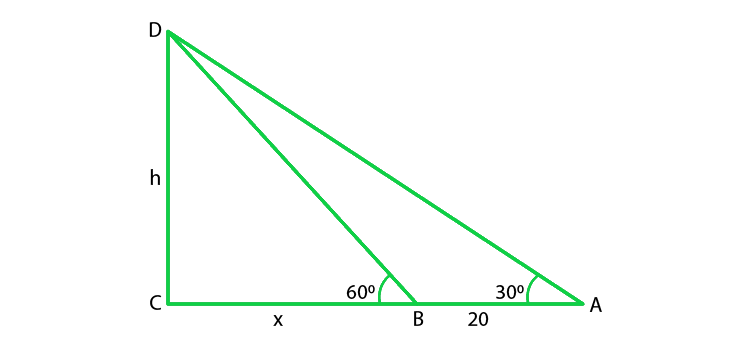Given that, the angle of elevation of top of the tower from point A(α) = 30°,

Angle of elevation of top of tower from point B(β) = 60°.

Distance between A and B, AB = 20 m

Let us assume that height of tower CD = ‘h’ m

and the distance between second point B from foot of the tower = ‘x’ m,

The given triangles are right angle triangles

So, ∠D = 90°

tan30o = h/(20 + x)

20 + x = h√3            —————–(i)

tanβ = CD/SD

tan60o = h/x

x = h√3                 ——————-(ii)

On Substituting eq(i) in (ii), we get

20 + h/√3 = h√3

h = 10√3

h = 17.31m

x = 10√3/√3

x = 10 m

So, the height of the tower = 17.32 m

Hence, the distance of the tower from point A = (20 + 10) = 30 m

### Question 17. From the top of a building 15 m high the angle of elevation of the top of tower is found to be 30°. From the bottom of the same building, the angle of elevation of the top of the tower is found to be 60°. Find the height of the tower and the distance between the building and the tower.

Solution: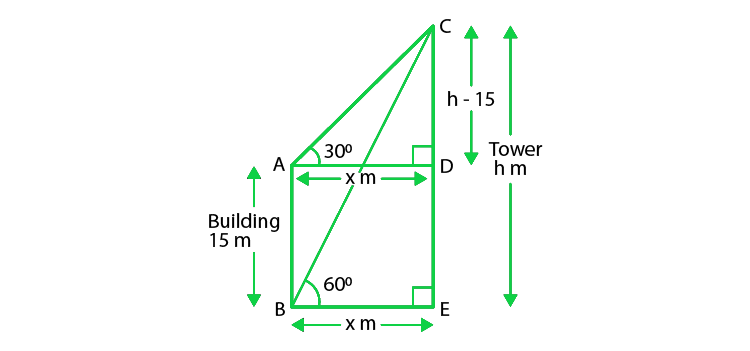Given that, the height of the building is AB = 15 m

Angle of elevation of top of the tower from top of the building(α) = 30°,

Angle of elevation of top of the tower from bottom of the building(β) = 60°,

Distance between the tower and the building BE = x,

Let us assume that the height of the tower CE = h

Here ABED is a rectangle
BE = AD = x cm

AB= DE = 15 cm

Now, In ΔCDA

tan30o = h – 15/x

x = h – 15√3          ——————-(i)

In ΔBEC

tanβ = CE/BE

tan60o = h/ x

x = h/ √3  ————–(ii)

From eq(i) and (ii), we get

h/ √3 = h – 15√3

h = 22.5

Now put the value of h in eq(ii), we get

x = 22.5/√3 = 12.990

Hence, height of the tower above ground is 22.5 m and

the distance between the building and the tower is 12.990

### Question 18. On a horizontal plane there is a vertical tower with a flag pole on the top of the tower. At a point 9 m away from the foot of the tower the angle of elevation of the top and bottom of the flag pole are 60° and 30° respectively. Find the height of the tower and the flag pole mounted on it.

Solution: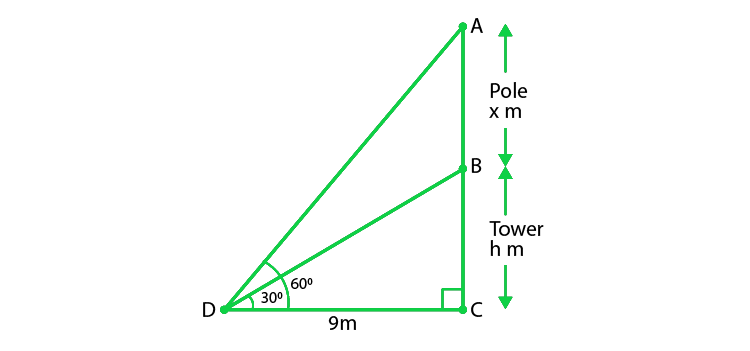Given that, the distance of the point of observation from foot of the tower BC = 9 m,

Angle of elevation of top of the pole(α) = 60°,

Angle of elevation of bottom of the pole(β) = 30°,

Let us assume that the height of the tower (BC) = h cm

And the height of the pole(AB) = x cm

From the figure we conclude that ΔACD and ΔBCD are right angle triangles

So, ∠C = 90°

In ΔACD,

tanα = AC/CD

tan60o = h + x / 9

h + x = 9√3                 ———————(i)

In ΔBCD

tanβ = BC/CD

tan30o = h / 9

h = 9/√3

h = 3√3            ——————-(ii)

h = 5.196 m

Now put the value of h in eq(1), we get

x = 9√3 – 3√3

x = 6√3

x = 10.392 m

Hence, the height of the tower is 5.196 m, and the height of the tower pole is 10.392 m.

### Question 19. A tree breaks due to storm and the broken part bends so that the top of the tree touches the ground making an angle of 30° with the ground. The distance between the foot of the tree to the point where the top touches the ground is 8 m. Find the height of the tree.

Solution: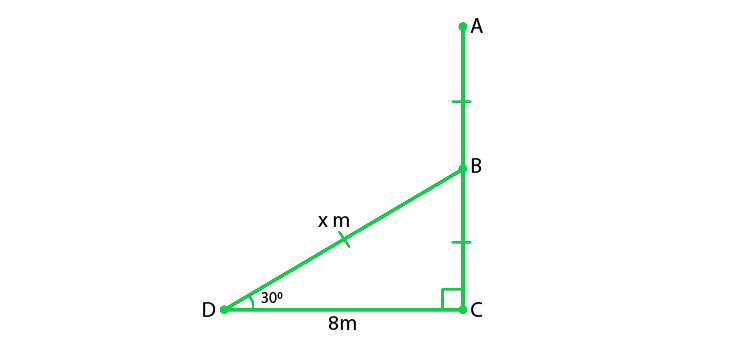Let us considered the initial height of the tree be AC.

And, due to storm the tree is broken at point B.

So, let us assume that the bent portion of the tree be AB = x m and the remaining portion BC = h m

So, the height of the tree AC = (x + h) m

And the distance between the foot of the tree to the point where the top touches the ground (DC) = 8m

Now, in ΔBCD

tan 30° = BC/DC

1/√3 = h/8

h = 8/√3

Again, in ΔBCD

cos30o = DC/BD

√3/2 = 8/x

x = 16/√3 m

So, x + h = 16/√3 + 8/√3

24/√3 = 8√3

Hence, the height of the tree is 8√3 m.

### Question 20. From a point P on the ground the angle of elevation of a 10 m tall building is 30°. A flag is hoisted at the top of the building and the angle of elevation of the flag staff from P is 45°. Find the length of the flag staff and the distance of the building from the point P.

Solution: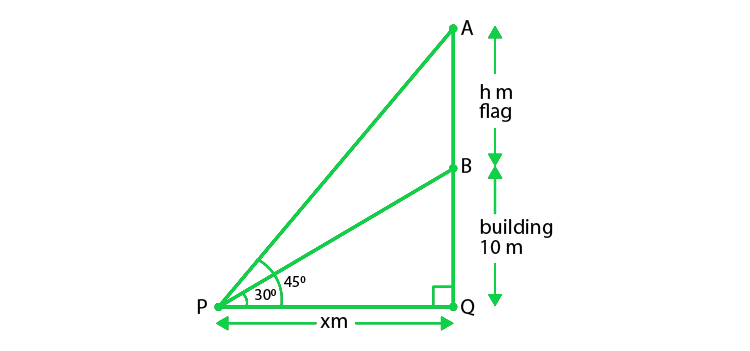Given that, the height of the building(BQ) = 10m

Angle of elevation of top of the building from P (α) = 30°,

Angle of elevation of top of flag staff from P (β) = 45°,

Let height of the flagstaff (AB) = h m,

From the figure we conclude that ΔAQP and ΔBQP are right angle triangles

So, ∠Q = 90°,

In ΔBQP,

tanα = BQ/QP

tan30o = 10/x

x = 10√3

x = 17.32 m ……(i)

In ΔAQP

tanβ = AQ/QP

tan45o = 10 + h /x

10 + h = x

Put the value of x from eq(i), we get

h = 17.32 – 10 m

h = 7.32 m

So, the height of the flag staff is 7.32 m, and

the distance between P and foot of the tower is 17.32 m

### (ii) properties of similar triangles

Solution: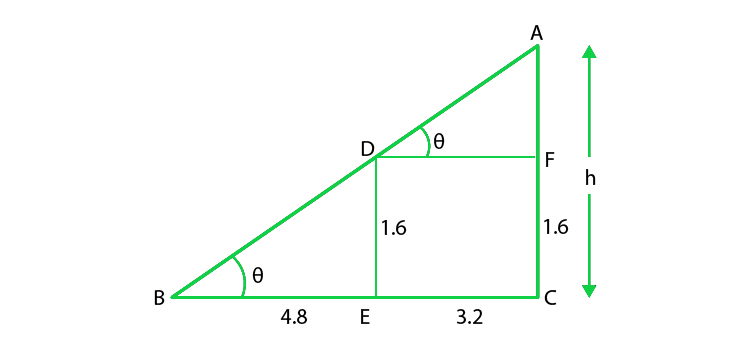Given that, A 1.6 m tall girl stands at a distance of 3.2 m

Let us considered AC be the lamp post of height = h cm

The height of the girl(DE) = 1.6m

Distance between the girl and lamp(EC) = 3.2m

and BE = 1.6 m

(i) In ΔBDE,

tan θ = 1.6/4.8

tan θ = 1/3

Next, In ΔABC

tan θ = h/ (4.8 + 3.2)

1/3 = h/8

h = 8/3 m

(ii) By using similar triangles,

ΔBDE and ΔABC are similar, we get

AC/BC = ED/BE

h / 4.8 + 3.2 = 1.6/4.8

h = 8/3 m

Hence, the height of the lamp post is is 8/3 m.

### Question 22. A 1.5 m tall boy is standing at some distance from a 30 m tall building. The angle of elevation from his eyes to the top of the building increases from 30° to 60° as he walks towards the building. Find the distance he walked towards the building.

Solution: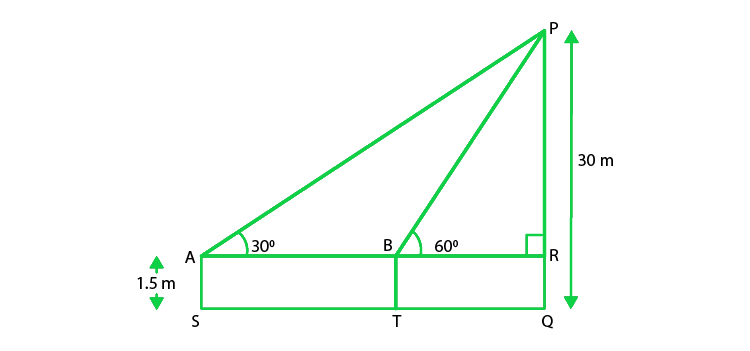Given that,

The height of the tall boy (AS) = 1.5 m,

The length of the building (PQ) = 30 m.

Let us considered the initial position of the boy be S.

And, then he walks towards the building and reached at the point T.

So,

AS = BT = RQ = 1.5 m,

PR = PQ – RQ = (30 – 1.5)m = 28.5 m,

In ΔPAR,

tan 30o = PR/AR

1/ √3 = 28.5/ AR

AR = 28.5√3

In ΔPRB,

tan 60o = PR/BR

√3 = 28.5/ BR

BR = 28.5/√3 = 9.5√3

So, ST = AB = AR – BR = 28.5√3 – 9.5√3 = 19√3

Hence, the distance which the boy walked towards the building is 19√3 m.

### Question 23. The shadow of a tower standing on level ground is found to be 40 m longer when Sun’s altitude is 30° than when it was 60°. Find the height of the tower.

Solution:

Let’ us considered AB be h m and BC be x m.

From the question, DC is 40 m longer than BC.

So, BD = (40 + x) m

And two right triangles ABC and ABD are formed.

In ΔABC,

tan 60o = AB/ BC

√3 = h/x

x = h/√3              —————(i)

In ΔABD,

tan 30o = AB/ BD

1/ √3 = h/ (x + 40)

x + 40 = √3h

h/√3 + 40 = √3h                  (using eq(i))

h + 40√3 = 3h

2h = 40√3

h = 20√3

Hence, the height of the tower is 20√3 m.

### Question 24. From a point on the ground the angle of elevation of the bottom and top of a transmission tower fixed at the top of 20 m high building are 45° and 60° respectively. Find the height of the transmission tower.

Solution: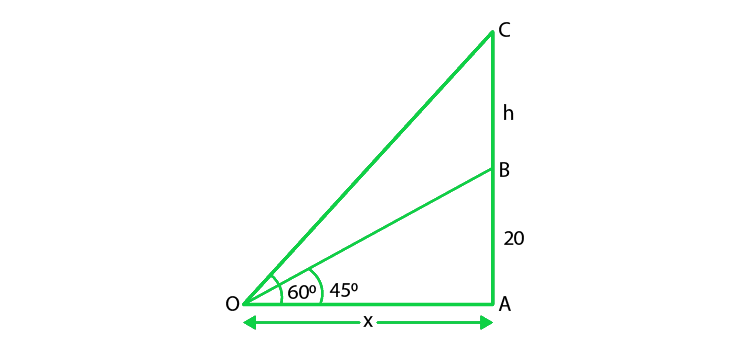Given that,

Height of the building(AB) = 20 m,

Let’ us considered height of tower above building(BC) = h,

Height of tower + building = (h + 20) m [from ground] = OA

Angle of elevation of bottom of tower(α) = 45°

Angle of elevation of top of tower(β) = 60°

Let us considered the distance between tower and observation point = x m

From the figure we conclude that ΔCAO and ΔBAO are right angle triangles

In ΔBAO

tanα = BA/OA

tan45o = 20/x

x = 20 m

Next, In ΔCAO

tanβ = CA/OA

tan60o = (h + 20) / x

h = 20(√3 – 1)

h = 20(1.73 − 1) = 20 x .732 = 14.64

Hence, the height of the tower is 14.64m

### Question 25. The angle of depression of the top and bottom of 8 m tall building from the top of a multistoried building are 30° and 45°. Find the height of the multistoried building and the distance between the two buildings.

Solution :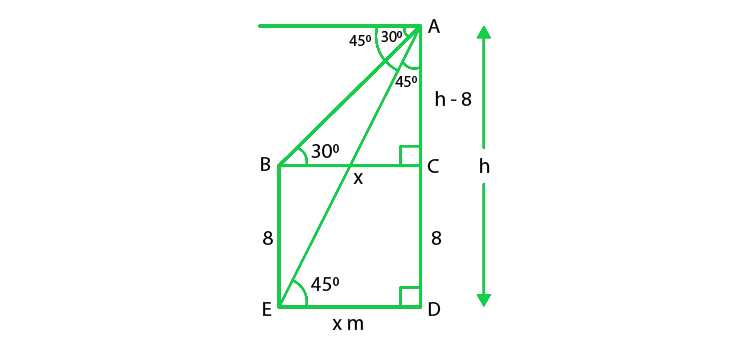Let us considered the height of multistoried building ‘h’ m = AD,

Height of the tall building = 8 m = BE,

Angle of depression of top of the tall building from the multistoried building = 30°,

Angle of depression of bottom of the tall building from the multistoried building = 30°,

And, let the distance between the two buildings = ‘x’ m = ED,

Therefore, BC = x and CD = 8 m

So, AC = (h – 8) m

We have,

In ΔBCA

tan 30o = AC/ BC

1/√3 = (h – 8)/x

x = √3(h – 8)              ……..(i)

1 = h/ x

h = x

h = √3(h – 8)                 (using eq(i))

h = √3h – 8√3

(√3 – 1)h = 8√3

h = 8√3/ (√3 -1)

On rationalizing the denominator by (√3 + 1), we get

h = 8√3(√3 + 1)/ (3 – 1)

h = 4√3(√3 + 1)

h = 12 + 4√3

= 4(3 + √3)

So, x = h = 4(3 + √3)

Hence, the height of the building is 4(3 + √3)m and

the distance between the building is 4(3 + √3)m

### Question 26. A statue 1.6 m tall stands on the top of a pedestal. From a point on the ground, angle of elevation of the top of the statue is 60° and from the same point the angle of elevation of the top of the pedestal is 45°. Find the height of the pedestal.

Solution: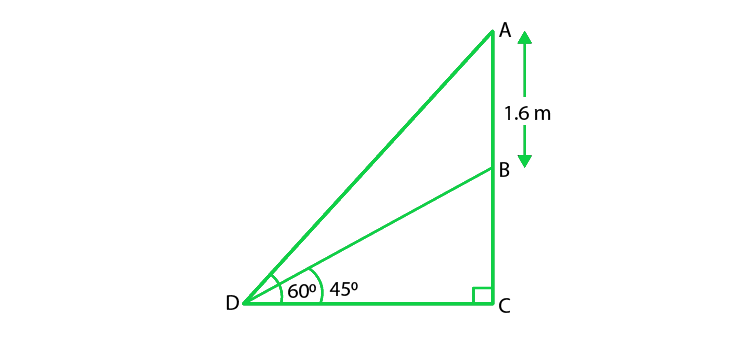Let us considered AB be the statue and BC be the pedestal and

D be the point on ground from where elevation angles are measured.

Given that,

Angle of elevation of the top of statue is 60°,

Angle of elevation of the top of the pedestal is 45°,

We have,

In ΔBCD,

tan 45° = BC/ CD

1 = BC/ CD

CD = BC

tan 60° = (AB + BC)/ CD

√3 = (AB + BC)/ BC  [Since CD = BC]

BC√3 = AB + BC

AB = (√3 – 1)BC

BC = AB/ (√3 – 1)

BC = 1.6/ (√3 – 1)

On rationalizing the denominator, we get

BC = 1.6(√3 + 1)/ 2

BC = 0.8(√3 + 1) m

Hence, the height of pedestal is 0.8(√3 + 1) m

My Personal Notes arrow_drop_up